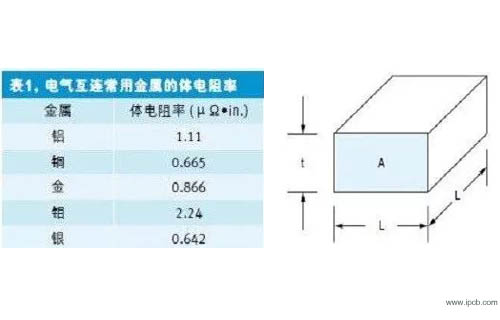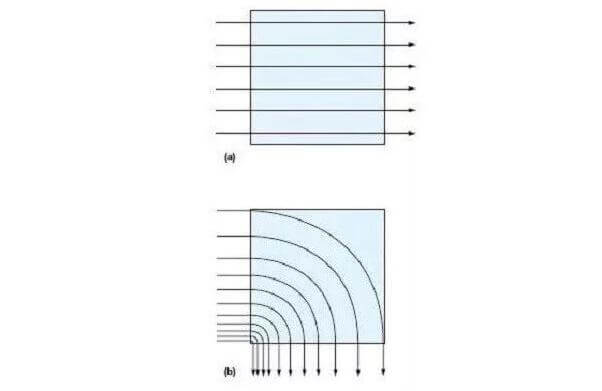전문 고주파 회로 기 판,고속 회로 기 판,IC 패 키 징 기 판,반도체 테스트 판,HDI 회로 기 판,소프트 하 드 결합 판,양면 다 층 판,PCB 디자인 및 PCBA 제조

### 빠 른 가격 획득.

sales@ipcb.com# PCB기술## PCB기술

Fast estimation of resistance value of PCB wiring

We usually need to quickly estimate the resistance of a wire or a plane on a printed circuit board, rather than make a tedious calculation. Although there are available printed circuit board layout and signal integrity calculation programs that can calculate the wiring resistance, we sometimes hope to adopt a fast and rough estimation method in the design process.

There is a way to do this easily, called "block statistics.". With this method, the resistance value (about 10%) of any geometry can be estimated in a few seconds. Once this method is mastered, the PCB area to be estimated can be divided into several blocks. After counting the number of all blocks, the resistance value of the whole wiring or plane can be estimated.Basic concepts

The key concept of block statistics is that the resistance value of square printed circuit board of any size (determined by thickness) is the same as that of other size blocks. The resistance value of the positive block only depends on the resistivity of the conductive material and its thickness. This concept can be applied to any type of conductive material. Table 1 shows some common semiconductor materials and their bulk resistivity.

For printed circuit boards, the important material is copper, which is the raw material for most circuit boards.

Let's start with the copper block in Figure 1. The length of the copper block is l, the width is l (because it is square), the thickness is t, and the cross-sectional area of the copper foil through which the current passes is a. The resistance of the copper block can be simply expressed as R = ρ L / A, where ρ is the resistivity of copper (this is the inherent property of the material, which is 0.67 μ Ω / in.) at 25 ℃.

Note, however, that section a is the product of length L and thickness t (a = LT). The L in the denominator and the L in the molecule cancel each other, leaving only r = ρ / T. Therefore, the resistance of copper block has nothing to do with the size of the block, it only depends on the resistivity and thickness of the material. If we know the resistance value of any size copper block, and can decompose the whole route to be estimated into multiple blocks, then we can add (count) the number of blocks to get the total resistance of the wire.

realization

In order to realize this technology, we only need a table, which gives the function relationship between the resistance value of a block on the printed circuit board trace and the thickness of copper foil. The thickness of copper foil is generally specified by the weight of copper foil. For example, 1 oz. copper means 1 oz. per square foot.

Table 2 gives the weights of four common copper foils and their resistivity at 25 ℃ and 100 ℃. Note that since the material has a positive temperature coefficient, the copper resistance increases with increasing temperature. For example, we now know that the resistance of a 0.5 oz. square copper foil is about 1 m Ω, which is independent of the size of the block. If we can decompose the printed circuit board wiring to be measured into several virtual blocks, and then add these blocks together, we can get the wiring resistance.

Let's take a simple example. Figure 2 shows a rectangular copper wire with a weight of about 0.5oz at 25 ℃, with a width of 1 inch and a length of 12 inches. We can decompose the routing into a series of squares, each of which is an inch long. So, there are a total of 12 squares. According to table 2, the resistance of each 0.5oz. Heavy copper foil block is 1m Ω. Now there are 12 blocks, so the total resistance of the wiring is 12m Ω.